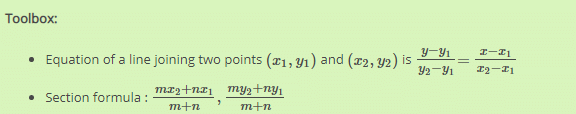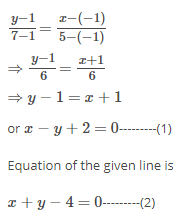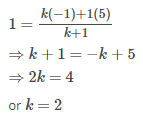MCQ: Straight Lines - Class 11

# MCQ: Straight Lines - Class 11 - Notes - Class 11

The line x+y =4 divides the line joining the points (1,-1) and (5,7) in the ratio?
Ref: https://edurev.in/question/620103/The-line-xy-4-divides-the-line-joining-the-points-1-1-and-5-7-in-the-ratio-

 1 Crore+ students have signed up on EduRev. Have you?The given points are (-1, 1) and (5,7).
Hence equation of the line joining the above points isThe point of intersection of the lines (1) and (2) is
xy=2x−y=−2
x+y=4
2x=2
⇒x=1 and y=3

Let the point (1,3) divide the line segment joining (-1,1) and (5,7) in the ratio 1 : k
By applying the section formula,Hence the line joining the points (-1, 1) and (5,7) is divided by line
x+y= in the ratio 1 : 2
The document MCQ: Straight Lines - Class 11 - Notes - Class 11 is a part of Class 11 category.
All you need of Class 11 at this link: Class 11
 Use Code STAYHOME200 and get INR 200 additional OFF

Track your progress, build streaks, highlight & save important lessons and more!

,

,

,

,

,

,

,

,

,

,

,

,

,

,

,

,

,

,

,

,

,

;